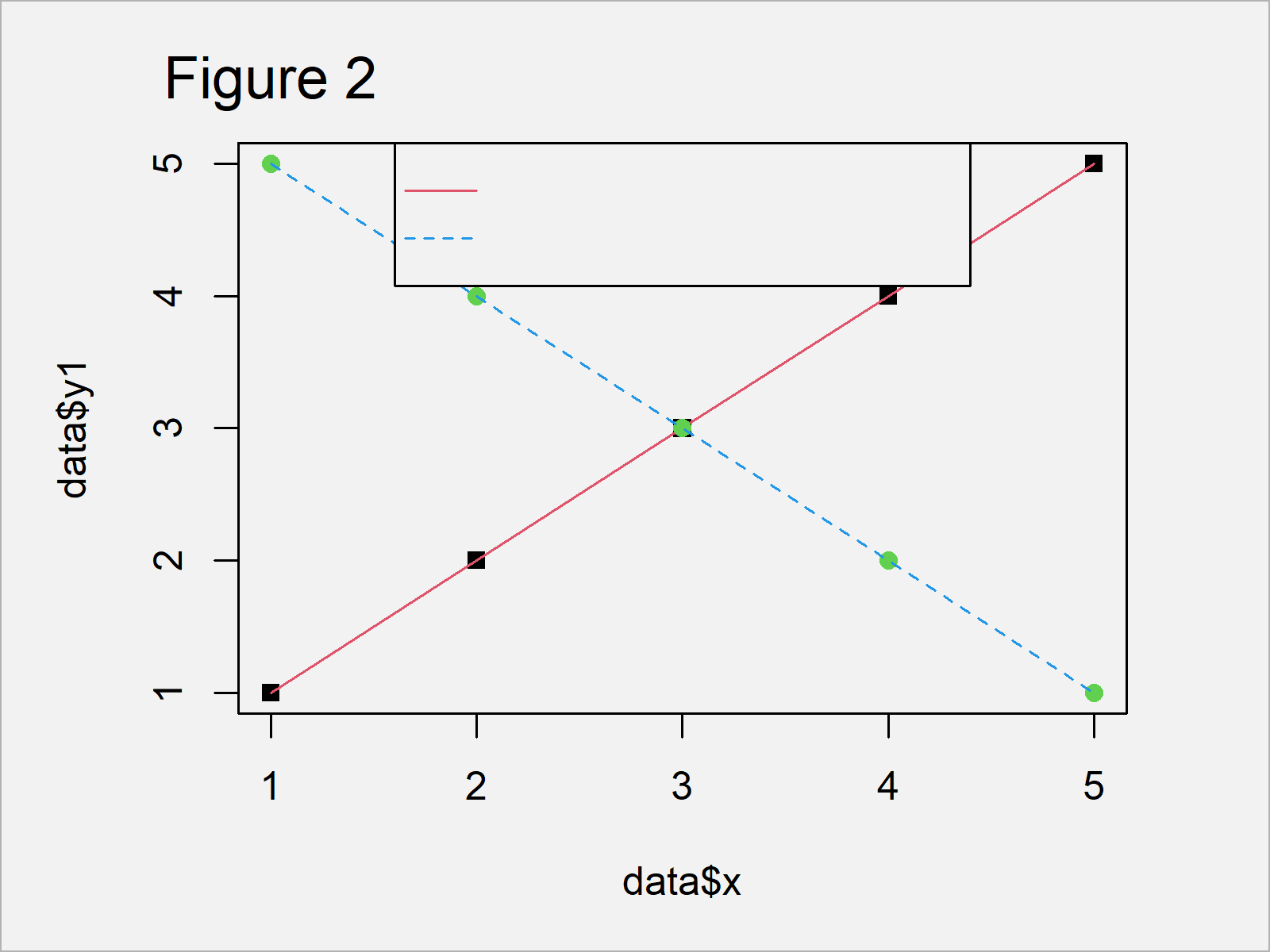# Different Colors of Points & Lines in Base R Plot Legend (Example)

In this R tutorial you’ll learn how to display the same legend item with different colors of points and lines.

The table of content looks like this:

So without further additions, here’s the step-by-step process:

## Creation of Example Data

As the first step, let’s create some example data in R:

```data <- data.frame(x = 1:5,                 # Create example data
y1 = 1:5,
y2 = 5:1)
data                                        # Print example data```Table 1 shows that our example data consists of five rows and three columns.

Let’s draw these data!

## Example: Legend with Different Points & Lines Using legend() Function Twice

The following R programming code illustrates how to create a Base R plot with a legend that displays points and lines in the legend with different colors.

As a first step, we have to draw our plot without legend:

```plot(data\$x, data\$y1, col = 1, pch = 15)    # Draw points & lines
lines(data\$x, data\$y1, col = 2, lty = 1)
points(data\$x, data\$y2, col = 3, pch = 16)
lines(data\$x, data\$y2, col = 4, lty = 2)```By running the previous R code we have managed to create Figure 1, i.e. a Base R graphic without any legend items.

As next step, we can add a legend to our plot using the legend() function. In the following R code, you have to note two things:

First, We are setting the text color to be equal to the background (i.e. white). Second, we are specifying that the points of our legend should not be shown yet (i.e. pch = c(NA, NA))

Let’s do this:

```legend(x = "top",                           # Add lines to legend
legend = c("Black Squares, Red Line",
"Green Points, Blue Line"),
text.col = "white",
lwd = 1,
col = c(2, 4),
lty = 1:2,
pch = c(NA, NA))```By running the previous R code we have created Figure 2, i.e. a plot with a legend that is just showing lines, but no text labels or points.

In the final step, we overlay another legend on top of the previously created legend. This time, we are adding text labels and points to the legend:

```legend(x = "top",                           # Add points to legend
legend = c("Black Squares, Red Line",
"Green Points, Blue Line"),
text.col = "black",
lwd = 1,
col = c(1, 3),
lty = c(0, 0),
pch = 15:16,
bty = "n")```As shown in Figure 3, we have created a Base R scatterplot with different colors in points and lines of the same legend items by executing the previous syntax.

## Video, Further Resources & Summary

Would you like to know more about legends in Base R? Then I can recommend watching the following video of my YouTube channel. I’m explaining the topics of this article in the video.

Besides that, you could have a look at the other tutorials of my website:

In this R tutorial you have learned how to modify lines and colors in legends. Please let me know in the comments, in case you have any additional questions.

Subscribe to the Statistics Globe Newsletter